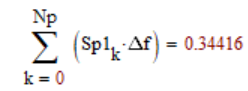cancel
Showing results for
Did you mean:
cancel
Showing results for
Did you mean:

Community Tip - Help us improve the PTC Community by taking this short Community Survey! X

## Problem with FFT9-Granite

## Problem with FFT

Hello,

I have a data that contains the position of a particle as a function of time. When I apply the fourier transform I do not get any frequencies however through the time position graph and the position histogram, I can see that the particle has a position around which it oscillates. I also need to calculate the power spectral density (PSD) as a function of frequency. Has anyone ever worked with something like that?

The original data is attached.

Thnak you.

Ulisses

12 REPLIES 1223-Emerald III
(To:M_U)

Something like this...?Success!

Luc23-Emerald I
(To:LucMeekes)

Unless I have forgotten, the first term in a CFFT is the steady value (equals the mean.)  The first frequency should then be zero,  And the magnitude (except for the first term) should be doubled.23-Emerald III
(To:Fred_Kohlhepp)
I took off the first element, kept the remainder of the first half of the spectrum data and doubled that.9-Granite
(To:LucMeekes)

Luc,

sorry for the delay of the answer because I was reading about it. I think that's right, but I'm analyzing the units on the vertical axis.

Thank you21-Topaz II
(To:M_U)

Hi,

This is all I can do with the data I have:21-Topaz II
(To:-MFra-)9-Granite
(To:-MFra-)

MF,

You suggested even more than I needed. I am still analyzing the details of your suggestion.

Thank you10-Marble
(To:M_U)

The particle does move back and forth about its average value (~82). However, it does so essentially randomly, with no single discernible frequency. Luc has done the essential calculation. However, there is a scale factor that needs to be applied in order to get the correct quantitative values for the power spectral density (PSD). For the signal p(t) with N samples, let P = CFFT(p). Sp1, the single sided PSD is defined for f=0 to fNyquist, and is given byThe factor of 2 sums the power for both positive and negative frequency components for f>0. Since all of the DFT routines, including CFFT, deal just with the data vectors, there is no information about the horizontal scale, be it time, distance, or something else. The N/fs factor provides the correct scaling (with CFFT) so that the result can be interpreted as a power spectral density. If the units of the data p are mm, then the PSD will have units of mm^2/Hz.

The plot shows my calc of PSD, a smoothed version, and a reference line of 1/f^2. The PSD follows the 1/f^2 behavior quite closely over the full frequency range. MCD v11 file and pdf attached.

Lou9-Granite
(To:LouP)

Loup,

sorry for the delay to answer because I was studying the subject. I think that's exactly what I needed. I'm analyzing the same data in KaleidaGraph and find something very close. I have a doubt,

the termshould converge to some specific value?

Thank you10-Marble
(To:M_U)

I don't see any anything in the attachment. Is it an image? Please try again.

Lou9-Granite
(To:LouP)

Lou,

the image is attached. Would you know the best method to integrate this spectrum?

Thank you

Ulisses10-Marble
(To:M_U)

Ulisses,

I don't know where you got the value for the sum of 0.344. In my sheet, (mathcad or pdf), I computed Sp1 as the spectral density of p0(t) - the original signal p(t) with the DC term removed.Just above the first time domain graph, the AC power (same as the variance) time domain calculation evaluates to 100.6...using the built-in var function. As a check, I did a summation of Sp1*df - effectively the frequency domain integral - just above the last log-log spectral plot. The summation evaluates to 100.6.., the same as the time domain calculation, as it should.

You ask "Would you know the best method to integrate this spectrum?" The summation is effectively the integration, since the spectrum is sampled and not continuous.

Does your value of 0.344 come from a worksheet you created? I don't see where it comes from.

Lou